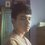# How many cubes

Recently I came across this problem from my friend in which I got stumped. Can anyone please help?

How many positive integer solutions $$(n, p)$$ are there to the equation $$n^3=p^2-p+1$$ where $$p$$ is a prime number?Note by Siam Habib
7 years, 3 months ago

This discussion board is a place to discuss our Daily Challenges and the math and science related to those challenges. Explanations are more than just a solution — they should explain the steps and thinking strategies that you used to obtain the solution. Comments should further the discussion of math and science.

When posting on Brilliant:

• Use the emojis to react to an explanation, whether you're congratulating a job well done , or just really confused .
• Ask specific questions about the challenge or the steps in somebody's explanation. Well-posed questions can add a lot to the discussion, but posting "I don't understand!" doesn't help anyone.
• Try to contribute something new to the discussion, whether it is an extension, generalization or other idea related to the challenge.

MarkdownAppears as
*italics* or _italics_ italics
**bold** or __bold__ bold
- bulleted- list
• bulleted
• list
1. numbered2. list
1. numbered
2. list
Note: you must add a full line of space before and after lists for them to show up correctly
paragraph 1paragraph 2

paragraph 1

paragraph 2

[example link](https://brilliant.org)example link
> This is a quote
This is a quote
    # I indented these lines
# 4 spaces, and now they show
# up as a code block.

print "hello world"
# I indented these lines
# 4 spaces, and now they show
# up as a code block.

print "hello world"
MathAppears as
Remember to wrap math in $$ ... $$ or $ ... $ to ensure proper formatting.
2 \times 3 $2 \times 3$
2^{34} $2^{34}$
a_{i-1} $a_{i-1}$
\frac{2}{3} $\frac{2}{3}$
\sqrt{2} $\sqrt{2}$
\sum_{i=1}^3 $\sum_{i=1}^3$
\sin \theta $\sin \theta$
\boxed{123} $\boxed{123}$

Sort by:

[EDITED]

$n^3 = p^2 - p +1$

$\Rightarrow n^3 - 1 = p^2 - p$

$\Rightarrow (n-1)(n^2+n+1)=p(p-1)$



Case 1: $p$ is a factor of $(n-1)$.

Let $\frac{n-1}{p} = k$;where $k$ is a natural number

$\Rightarrow n-1 = pk$

Now, $\frac{(n-1)}{p} (n^2+n+1) = p-1$

$\Rightarrow k(n^2+2+n-1) = p-1$

$\Rightarrow k(n^2+2+pk) = p-1$

$\Rightarrow kn^2 + 2k + pk^2 = p-1$

$\Rightarrow kn^2 + 2k + 1 = p-pk^2$

$kn^2+2k+1=p(1-k^2)$

$(kn^2+2k+1) > 0$. So, $(1-k^2) > 0$ or $-1. There exist no such value for $k$ which satisfies the equation as $k$ is a natural number.



Case 2:$p$ is a factor of $(n^2+n+1)$.

I'm still working on it.

- 7 years, 3 months ago

That is not true, is it? Try $(n, p)=(7, 19)$.

Can you find out what's wrong with your reasoning?

- 7 years, 3 months ago

I was totally wrong. :(

- 7 years, 3 months ago

It's only true that $p$ must divide one of the two factors

- 7 years, 3 months ago

- 7 years, 3 months ago

what if n-1 = kp or $n^{2} + n+ 1$ = kp , then you cannot assert that p = n-1 or $n^{2} + n+ 1$ like mursalin habib's case where kp = $n^{2} + n+ 1$ with k =3 ?

- 7 years, 3 months ago

Try rewriting it as $(n-1)(n^2+n+1) = p(p-1)$

- 7 years, 3 months ago

I tried that and considered it from multiple angle but, failed to go anywhere from there. Can you be more specific about what to do from there?

- 7 years, 3 months ago

I haven't seen a full answer yet. Mine is kind of ugly but I think it works.

As in the previous solutions, reduce to the case where $p | (n^2+n+1)$. Write $pk = n^2+n+1$, so $p-1 = k(n-1)$. Solve for $p$ to get $p = k(n-1) + 1$. Plug in to get $k^2(n-1) + k = n^2+n+1$. Write as a quadratic in $n$: $n^2 + (1-k^2) n + (k^2-k+1) = 0$.

Use the quadratic formula: $n = \frac12\left( k^2-1 \pm \sqrt{(k^2-1)^2-4(k^2-k+1)} \right) = \frac12 \left( k^2-1 \pm \sqrt{(k^2-3)^2+4k-12} \right)$.

If $k = 1$ or $k = 2$ then the quantity under the square root is negative. If $k = 3$ then we get $n = \frac12(8 \pm 6) = 1,7$. The former solution is no good ($p = 1$) but the latter solution yields $(7,19)$. If $k \ge 4$ then the quantity inside the square root is greater than $(k^2-3)^2$. But since $k^2-k+1 > 0$ for all $k$, the quantity under the square root is less than $(k^2-1)^2$. So the square root itself is strictly between $k^2-3$ and $k^2-1$.

So if we choose the minus sign for $n$, we get that $n$ is strictly between $0$ and $1$, which is no good. If we choose the plus sign for $n$, we get that $n$ is strictly between $k^2-2$ and $k^2-1$, which is also no good. So $(7,19)$ is the only solution.

- 7 years, 3 months ago

Thank you very much. It was great help. I have been trying this problem for almost two weeks. You are awesome.

- 7 years, 3 months ago